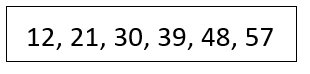Mathematics
Easy

Question

# This increasing number pattern was made using a rule.The rule that could have been used to make this pattern isHint:

### The given sequence is 12, 21, 30, 39, 48, 57We have to guess the rules used to write the above sequence.Let’s check the options one by one. We will perform the operations and check if it matches the sequence.Option A : Multiply by 3Let’s take the first term of the sequence and start the operations.12 × 3 = 36It is not the second term of the above sequence. So, option A is wrong.Option B : Add 712 + 7 = 19It is not the second term of the above sequence. So, option B is wrong.Option C : Multiply by 1012 × 10 = 120It is not the second term of the above sequence. So, option C is wrong.Option D : Add 912 + 9 = 2121 + 9 = 3030 + 9 = 3939 + 9 = 4848 + 9 = 57So, the sequence becomes 12, 21, 30, 39, 48, 57. It matches the given sequence.Therefore, option D is the right option.

For such questions, we should know the basic operations. When the rule used to write the pattern is not mentioned, we have to use all four operations to check the pattern. The operations are addition, subtraction, division, and multiplication.

### Related Questions to study#### With Turito Foundation.#### Get an Expert Advice From Turito.